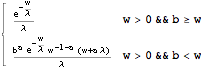## Maximum of:       Triangular, Uniform and half-Halo New in mathStatica 2.0

Consider three different distributions defined over three different domains of support …

Letwith pdf   f(x),        let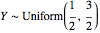with pdf g(y),      and let Z half-Halo with pdf h(z):

In:=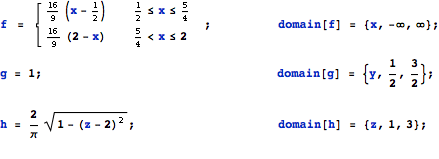Here are the three pdf’s illustrated: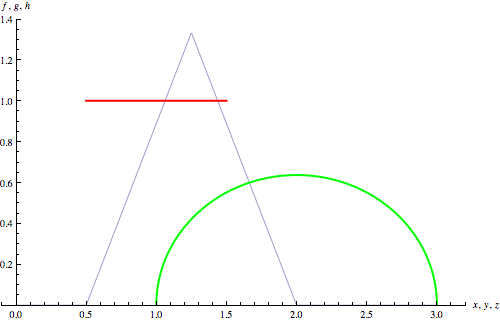Suprematism No. 1:    f(·) Triangular      g(·) Uniform        h(·) half-Halo

We seek the pdf of W = max(X, Y, Z) . The solution pdf is simply:

In:=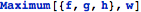Out:=Here is a plot of the pdf of the maximum, together with the underlying pdf’s:Suprematism No. 2:  The Malevich Maximum —  pdf of the maximum, together with the 3 underlying pdf’s## Maximum of:       Six different Uniform random variables New in mathStatica 2.0

Letwith pdf f(x):

In:=Here are the six pdf’s corresponding to b = 1, 2, 3, 4, 5 and 6:

In:=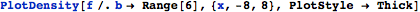Out=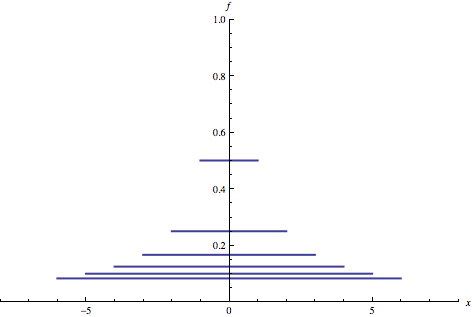Problem: Find the pdf of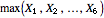.

Solution: The solution pdf is simply:

In:=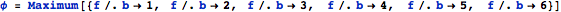Out=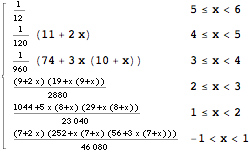with domain of support:      (we define piecewise functions over the real line)

In:=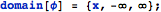Here is a plot of the solution pdf:

In:=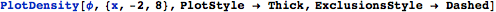Out=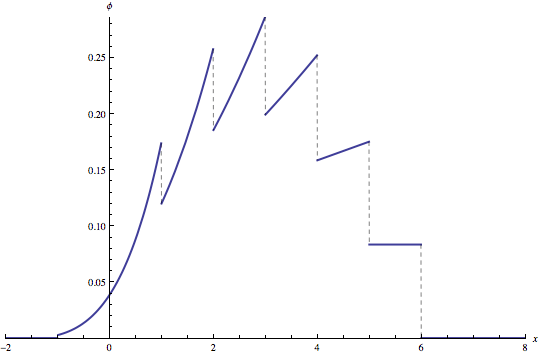A quick Monte Carlo 'check’ of the exact solution we have just plotted:
We first generate 6 pseudo-random data sets corresponding to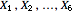, and each containing 250000 pseudo-random drawings:

In:=Next, we transpose from 6 data sets each of size 250000  … to … 250000 samples each of size 6. Each sample of 6 represents a single pseudo-random drawing from. Then, we map the Max function across each sample of 6, generating our 250000 empirical drawings of the sample maximum:

In:=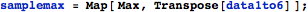We can now make a frequency plot to compare the pseudo-random Monte Carlo solution () with the theoretical symbolic solution φ(x) () derived above:

In:=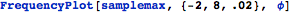Out=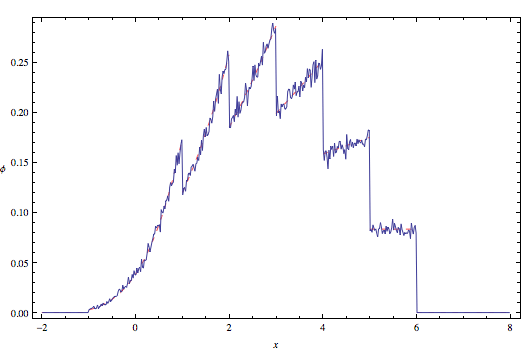For the win!## Minimum of:       Pareto and Exponential New in mathStatica 2.0

Let X ∼ Pareto(a, b)  with pdf f(x):

In:=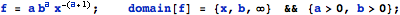and let Y ∼ Exponential(λ) with pdf g(y):

In:=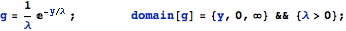Problem:
Find the pdf of W = min(X, Y).

Solution: The solution is simply:

In:=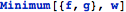Out=